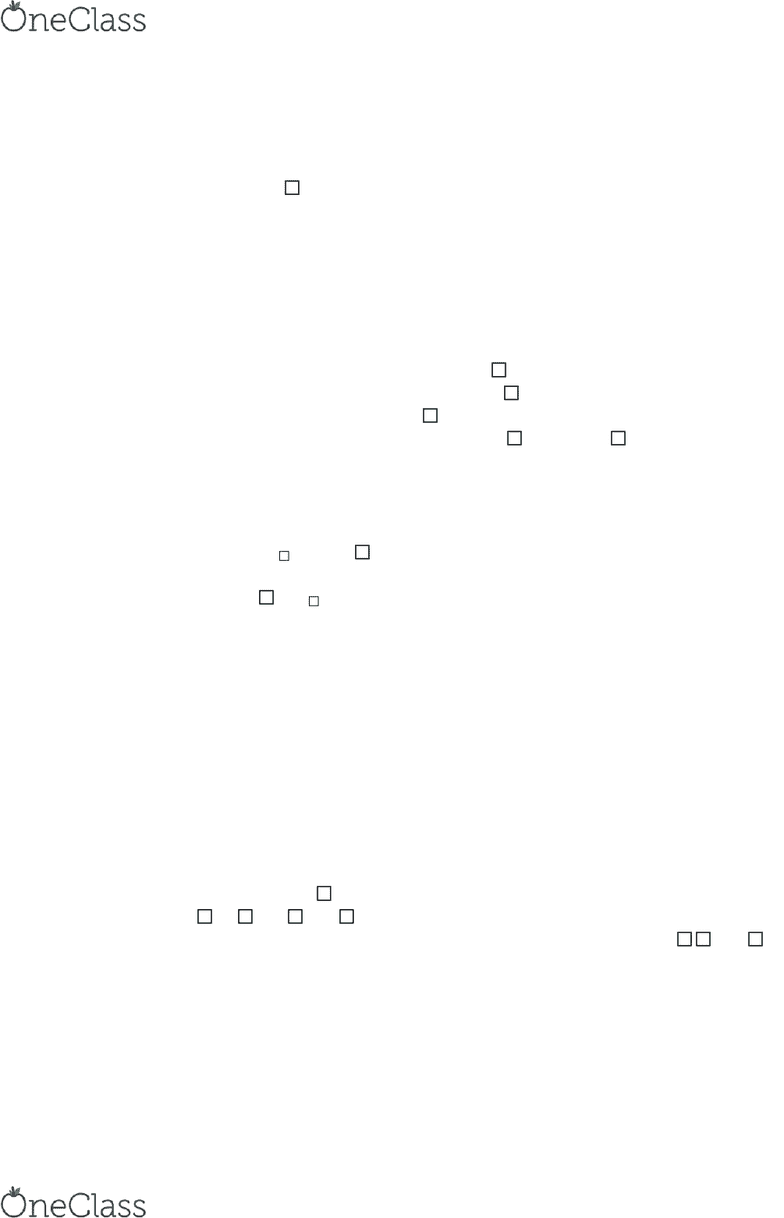Textbook Notes (290,000)
US (110,000)
UM (1,000)
PHY (20)
PHY 101 (10)
Chapter 11

# PHY 101 Chapter Notes - Chapter 11: Point Particle, Angular Momentum, Right-Hand Rule

Department
Physics
Course Code
PHY 101
Professor
Dr.sharon Zane
Chapter
11

This preview shows page 1. to view the full 4 pages of the document.11-1 Torque
The tendency for a force to cause a rotation increases with
the distance, r, from the axis of rotation to the force
Torque: = rF; units: N*m; torque is the tangential force, F
is the magnitude of the applied force (only when applied on
tangential)
The above equation is only for tangential forces
If the force is applied in the radial direction along the axis of
rotation, the axle on which the object turns will produce an
equal and opposite force and it will remain at rest
If the force is applied an angle to the radial line, first
resolve it into components; Fcos is the radial component
and therefore zero, Fsin is the tangential component
General definition of Torque: = r(Fsin ); units: N*m
The torque can also be defined as the cross product
between the radius and force vectors
Moment arm: the perpendicular distance from the axis of
rotation to the line of the force (line draw through the force
vector) r = rsin
The torque can also be written as the moment arm times the
force: = r F
A torque applied to an object produces an angular
acceleration; if a torque acts on an object at rest it will start
rotating and if it acts on a rotating object it will change its
velocity
Torque>0 if it causes counterclockwise angular acceleration;
T<0 if the torque causes a clockwise angular acceleration
W/ more than one torque in a system, the sign of each is
determined by the angular acceleration it alone produces
If the sum of 2+ torques is positive, acceleration is
counterclockwise; if negative clockwise
11-2 Torque and Angular Acceleration
A single torque acting on an object causes it to have angular
acceleration
= /I; = I
Newton’s Second Law for Rotational Motion: = I
11-3 Zero Torque and Static Equilibrium
Simply because the forces on an object sum to zero does not
guarantee the object remains at rest
For an object to remain completely at rest (no translation or
rotation) two conditions are imposed
The net force acting on the object must be zero so that
there is no translational acceleration
find more resources at oneclass.com
find more resources at oneclass.com